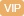| APP | 校园号 | 客服 客服热线：400-863-9889

400-863-9889（2018春•获嘉县月考）直接写出得数．
 600÷2= 420÷2= 10×57= 120×4= 360÷3= 13×20= 40×21= 13×40= 0.6+0.9= 0.8-0.7= 1.9+0.4= 1.6-0.8=
【考点】整数的乘法及应用整数的除法及应用小数的加法和减法【专题】计算题．

【解答】解：教室中黑板面积大约是 平方，
240毫=0.24；
6小时=1.5．

【点评】此题考根据情景选择合计量单位，要注意系生活计量单位和数据的小，活的选择；还考名数的换算，把高级单位的名数换算成低级单位的名数就乘单位进率，低级单位的算成高级位的名数，就除以间率．

0/0